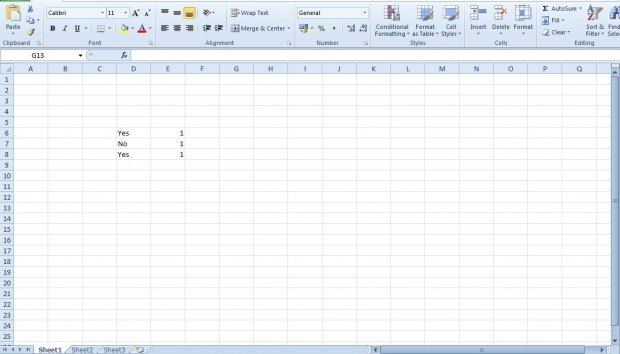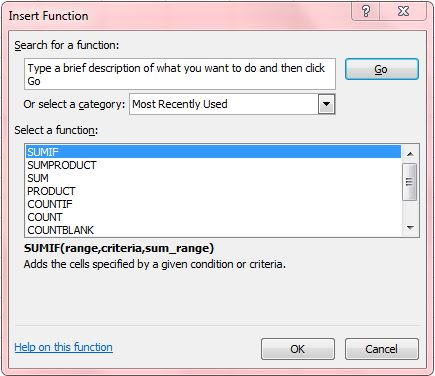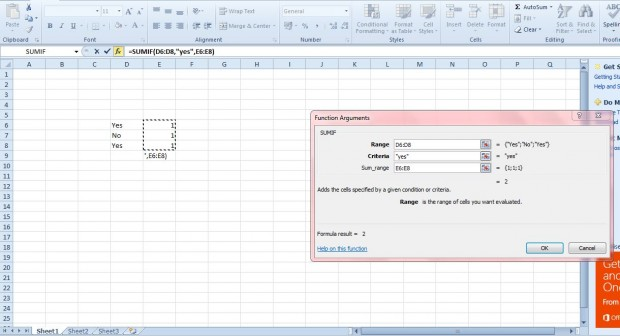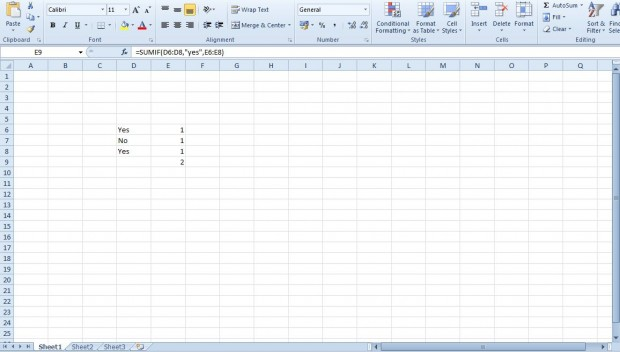# How to add the SUMIF function to spreadsheets in Excel 2010/13 [Tip]

The basic SUM function in Excel is sufficient for adding a variety of numbers across a range of cells. However, what if you only need to add a few numbers that match a specific criteria from a range of cells? If that is so, add the SUMIF function to your spreadsheet.

First, enter a few numbers in one column of your Excel spreadsheet. As a basic example enter one in three column cells. Then enter yes in a couple of cells next to them and no in another as shown in the shot below. Yes and no are to be the criteria for summing the numerical cells together.Now select a cell on the spreadsheet to include the SUMIF function in, and press the (fx) Insert Function button. That will open the window in the shot directly below.Next, select the SUMIF function from that window. Press the OK button to open the window below in which you can select the cells to include the SUMIF function. Select the column that includes the yes/no criteria for the Range box. Enter yes in the Criteria box. Then select the column that includes the numbers for the Sum_range box as below.Now press the OK button on the window. The spreadsheet will then sum those cells in the same row as the Yes criteria as show below. As such, that amounts to two in the example below. The function for that is =SUMIF(D6:D8,”yes”,E6:E8).So with this function you can now add cells based on more specific criteria. It could be handy for a variety of account spreadsheets.